价值13000svip视频教程,前端大神匠心打造,零基础前端开发工程师视频教程全套,基础+进阶+项目实战,包含课件和源码

出租广告位,需要合作请联系站长

+关注

2021-05(12)

2021-06(108)

2021-07(4)

2021-08(49)

2021-09(67)

# 第4章 表达式与操作符

## 4.1 主表达式

``````1.23           //数值字面量
"hello"        //字符串字面量
/pattern/      //正则表达式字面量
``````

JavaScript的一些保留字也是主表达式：

``````true           //求值为布尔值true
false          //求值为布尔值false
null           //求值为null值
this           //求值为当前对象
``````

``````i             //求值为变量i的值
sum           //求值为变量sum的值
undefined     //全局对象undefined属性的值
``````

## 4.2 对象和数组初始化程序

``````let matrix=[[1,2,3],[4,5,6],[7,8,9]]
``````

``````let p={x:2.3,y:-1.2}     //有两个属性的对象
let q={}                 //没有属性的空对象
q.x=2.3;q.y=-1.2;        //现在q拥有了跟p一样的属性
``````

``````let rectangle={
upperLeft:{x:2,y:2},
lowerRight:{x:4,y:5}
};
``````

## 4.4 属性访问表达式

``````expression.identifier
expression[expression]
``````

eg:

``````let o={x:1,y:{z:3}}
let a=[o,4,[5,6]]
o.x
=>1
o.y.z
=>3
o["x"]
=>1
a
=>4
a["1"]
=>6
a.x
=>1
``````

### 4.4.1 条件式属性访问

ES2020增加了两个新的属性访问表达式：

``````expression?.identifier
expression?.[expression]
``````

``````let a={b:null};
a.b?/.c.d
=>undefined
``````

``````let a;              //忘记初始化这个变量了！
let index=0
try{
a[index++];      //抛出TypeError
}catch(e){
index            //抛出TypeError之前发生了递增
}
=>1
a?.[index++]
=>undefined         //因为a是undefined
index
=>1
a[index++]          //不能索引undefined
=>VM4584:1 Uncaught TypeError: Cannot read property '1' of undefined
at <anonymous>:1:2
(anonymous) @ VM4584:1
``````

## 4.5 调用表达式

``````f(0)                   //f是函数表达式，0是参数表达式
Math.max(x,y,z)        //Math.max是函数。x、y、z是参数
a.sort()               //a.sort()是函数，没有参数
``````

### 4.5.1 条件式调用

``````function square(x,log){  //第二个参数是一个可选的函数
if(log){         //如果传入了可选的函数
log(x);      //调用这个函数
}
return x*x;      //返回第一个参数的平方
}
``````

``````function square(x,log){   //第二个参数是一个可选的函数
log?.(x);         //如果有定义则调用
return x*x;
}
``````

``````let f=null,x=0；
try{
f(x++);      //因为f是null所以抛出TypeError
}catch(e){
x           //抛出异常前发生了递增
}
=>1
f?.(x++)
=>undefined     //f是null，但不会抛出异常
x
=>1             //因为短路，递增不会发生
``````

``````o.m()        //常规属性访问，常规调用
o?.m()       //条件式属性访问，常规调用
o.m?.()      //常规属性访问，条件式调用
``````

## 4.6 对象创建表达式

``````new Object()
new Point(2,3)
``````

``````new Object
new Date
``````

## 4.7 操作符概述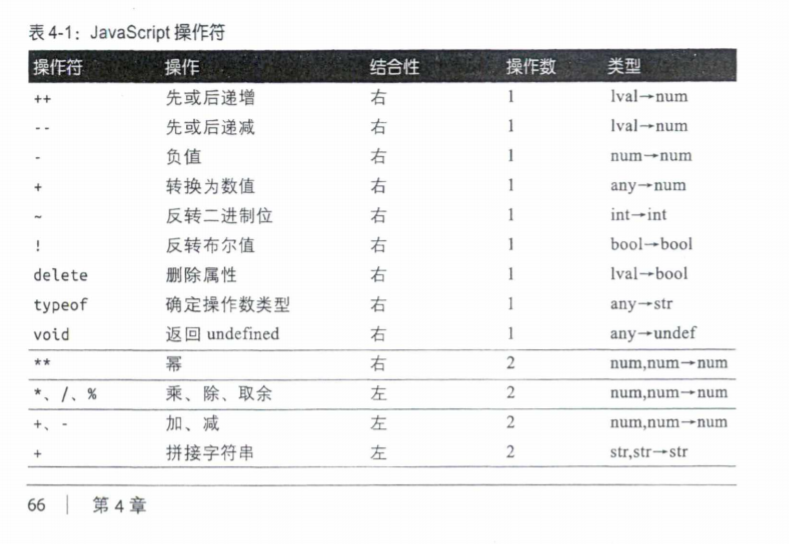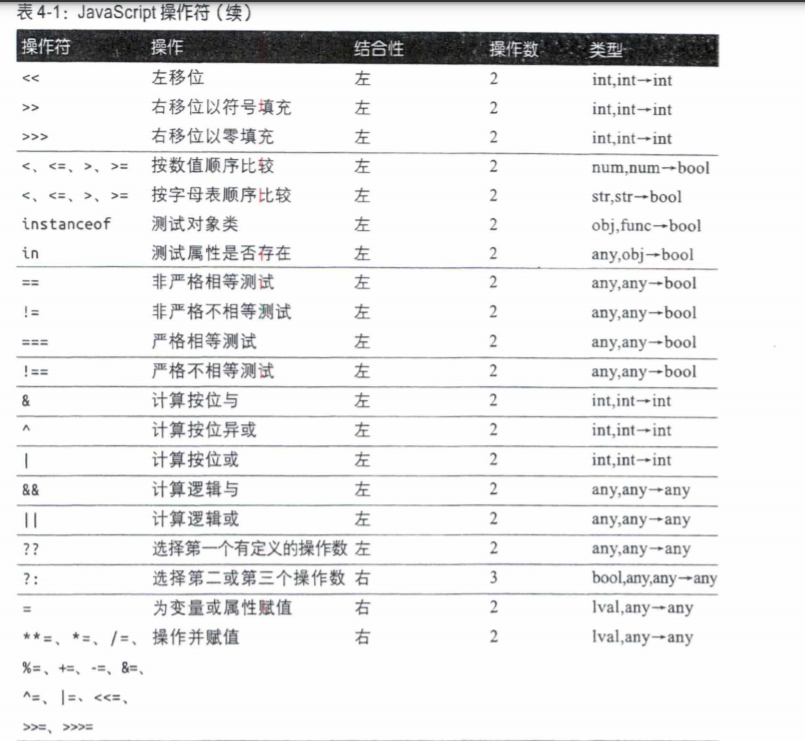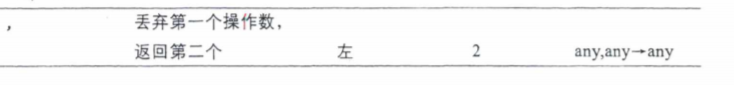### 4.7.2 操作数与结果类型

JavaScript操作符通常会按照需要转换操作数的类型。比如，乘法操作符*期待数值参数，而表达式"3" * “5"之所以合法，是因为JavaScript可以把操作数转换为数值。因此这个表达式的值是数值15，而非字符串"15”。也要记住，每个JavaScript值要么是“真值”要么是“假值”，因此期待布尔值操作数的操作符可以用于任何类型的操作数。

### 4.7.4 操作符优先级

``````w=x+y*z;
``````

``````w=(x+y)*z
``````

``````//my是一个由function属性的对象，function属性
//是一个函数的数组。这里调用了x号函数，并传给它
//参数y，然后再求值函数调用返回值的类型
typeof my.functions[x](y)
``````

JavaScript新增的操作符并不总是符合这个优先级模式。比如再表4-1中，??操作符比||和&&优先级低，而实际上它相对于这两个操作符的优先级并没有定义，ES2020要求混用??和||或&&时必须使用圆括号。类似地，新的幂操作符**相对于一元负值操作符也没有明确定义，因此在同时求负值和求幂时也必须使用圆括号

### 4.7.5 操作符结合性

``````w=x-y-z;
``````

``````w=((x-y)-z);
``````

``````y=a**b**c;
x=~-y;
w=x=y=z;
q=a?b:c?d:e?f:g;
``````

``````y=(a**(b**c));
x=~(-y);
w=(x=(y=z))
q=a?b:(c?d:(e?f:g))
``````

## 4.8 算术表达式

** 操作符的优先级高于*、/和%（后三个的优先级又高于+和-）。与其他的操作符不同， ** 具有右结合性，即2 ** 2 ** 3相对于2 ** 8而非4 ** 3。另外，-3 ** 2这样的表达式本质上是有歧义的，取决于一元减号和幂操作的相对优先级，这个表达式可能意味着(-3) ** 2，也可能意味着-(3**2)。对于这种情况，不同语言的处理方式也不同。JavaScript认为这种情况下不写括号就是语法错误，强制你自己消除表达式的歧义。 **是JavaScript中最新的操作符，是ES2016中增加的。但Math.pow()函数在JavaScript很早的版本中就有了，它与 ** 操作符执行完全相同的操作。

/操作符用第二个操作数除第一个操作数。如果你习惯了区分整数和浮点数的编程语言，应该自带整数相除得到整数。但在JavaScript中，所有数值都是浮点数，因此所有除法操作得到的都是浮点数，比如5/2得到的是2.5而不是2。被0除得到正无穷或负无穷，而0/0求值为NaN。这两种情况都不是错误。

%操作符计算第一个操作数对第二个操作数取模的结果。换句话说，它返回第一个操作数被第二个操作数整除之后的余数。结果的符号与第一个操作数相同。例如，5%2求值为1，而-5%2求值为-1；

``````6.5%2.1
0.19999999999999973
``````

### 4.8.1 +操作符

``````1+2                   //=>3
"hello"+" "+"there"   //=>"hello there"
"1"+"2"               //=>"12"
``````

• 如果有一个操作数是对象，则+操作符使用3.9.3节介绍的对象到原始值的算法把该对象转换成原始值。Date对象用toString()方法来转换，其他所有对象通过valueOf()转换（如果这个对象返回原始值）。不过，多数对象并没有valueOf()方法，因此它们也会通过toString()方法转换。
• 完成对象到原始值的转换后，如果有操作数是字符串，另一个操作数也会被转换为字符串并进行拼接。
• 否则，两个操作数都被转换成数值（或NaN），计算加法。

``````1+2
=>3               //加法
"1"+"2"
=>"12"            //拼接
"1"+2
=>"12"            //数值转换成字符串后再拼接
1+{}
=>"1[object Object]"       //对象转换为字符串后再拼接
true+true
=>2              //布尔值转换为字符串后计算加法
2+null
=>2              //null转换为0后计算加法
2+undefined
=>NaN            //undefined转换为NaN后计算加法
``````

``````1+2+" blind mice"
=>"3 blind mice"
1+(2+" blind mice")
=>"12 blind mice"
``````

### 4.8.2 一元算术操作符

一元操作符将其操作数转为数值（或NaN）并返回转换后的值。如果操作数是数值，则它什么也不做。由于BigInt值不能转换为常规数值，因此这个操作符不应该用于BigInt。

当-用作一元操作符时，它在必要时将操作数转换为数值，然后改变结果的符号。

++操作符递增其操作数（也就是加1），这个操作数必须是一个左值（变量、数组元素或对象属性）。这个操作符将其操作数转换为数值，在这个数值上加1，然后将递增后的数值再赋值会这个变量、元素或属性。

==++操作符的返回值取决于它与操作数相对位置。如果位于操作数前面，则可以称其为前增操作符，即先递增操作数，再求值为操作数递增后的值。如果位于操作数后面，则可以称其为后递增操作符，即它也会递增操作数，但仍然求值为该操作数未递增的值。==看看下面两行代码，注意它们的差异：

``````let i=1,j=++i;
i
=>2
j
=>2
let n=1,m=n++;
n
=>2
m
=>1
``````

--操作符也期待左值操作数。它会将这个操作数转换为数值，减1,然后把递减后的值再赋值给操作数。与++操作符类似，–返回的值取决于它与操作数的相对位置。如果位于操作数前面，它递减并返回递减后的值。如果位于操作数后面，它递减操作数，但返回未递减的值。在位于操作数后面时，操作数与操作符之间不能有换行符。

## 4.9 关系表达式

### 4.9.1 相等与不相等操作符

== 和 === 操作符分别用两个相同的标准检查两个值是否相同。这两个操作数都接受任意类型的操作数。都在自己的操作数相同时返回true，不同时返回false。=== 操作符被称为严格相等操作符（或者全等操作符），它根据严格相同的定义检查两个操作数是否“完全相同”。 == 操作符被称为相等操作符，它根据更宽松的（允许类型转换的）相同定义检查两个操作数是否“相等”。

!= 和 !== 操作符测试的关系与 == 和 === 恰好相反。!=不相等操作符在两个值用 == 测试相等时返回false，否则返回true。!==操作符在两个值严格相等时返回false，否则返回true。

#### 严格相等

• 如果两个值类型不同，则不相等。
• 如果两个值都是null或都是undefined，则相等、
• 如果两个值都是布尔值true或都是布尔值false，则相等。
• 如果一个或两个值是NaN，则不相等（虽然有点意外，但NaN确实不等于如何其他值，也包括NaN本身！要检查某个值是不是NaN，使用x!==x或全局isNaN()函数）
• 如果两个值都是数值且值相等，则相等。如果一个值是0而另一个是-0，则也相等。
• 如果两个值是字符串且相同位置包含完全相等的16位值，则相等。如果两个字符串长度或内容不同，则不相等。两个字符串可能看起来相同，也表示同样的意思，但底层编码却使用不同的16位值序列。JavaScript不会执行Unicode归一化操作，像这样的两个字符串用 === 或 == 操作符都不会判定相等。
• 如果两个值引用同一个对象、数组或函数，则相等。如果它们引用不同的对像，即使两个对象有完全相同的属性，也不相等。

#### 基于类型转换的相等

• 如果两个值类型相同，则按照前面的规则测试它们是否严格相等。如果严格相等，则相等。否则不相等。
• 如果两个值类型不同，== 操作符仍然认为它们相等。此时它会使用一下规则，基于类型转换来判定相等关系。
- 如果一个值是null，另一个值是undefined，则相等。
- 如果一个值是数值，另一个值是字符串，把字符串转换成数值，再比较转换后的数值。
- 如果一个值为true，把它转换为1，再比较。如果有一个值为false，把它转换为0，再比较。
- 如果一个值是对象，另一个值是数值或字符串，先使用3.9.3描述的算法把对象转化成原始值，再比较。JavaScript内置的核心类先尝试使用valueOf()，再尝试使用toString()。但Date是个例外，这个类执行toString()转换。
- 其他任何值的组合都不相等。

### 4.9.2 比较操作符

<操作符在第一个操作数小于第二个操作数是求值为true，否则求值为false。

>操作符在第一个操作数大于第二个操作数时求值为true，否则求值为false。

<=操作符在第一个操作数小于等于第二个操作数时求值为true，否则求值为false。

>=操作符在第一个操作数大于等于第二个操作数时求值为true，否则求值为false。

• 如果有操作数求值为对象，该对象会按照3.9.3节的描述被转换为原始值。即如果它的valueOf()方法返回原始值，就使用这个值，否则就使用它的toString()方法返回的值。
• 如果在完成对象到原始值的转换后两个操作数都是字符串，则使用字母表顺序比较这两个字符串，其中“字母表顺序”就是组成字符串的16位Unicode值的数值顺序。
• 如果在完成对象到原始值的转换后至少有一个操作数不是字符串，则两个操作数都会被转换为数值并按照数值顺序来比较。0和-0被认为相等。Infinity比它本身之外的任何数都大，-Infinity比它本身的任何值都小。如果有一个操作数是（或转换后是NaN），则这些操作符都返回false。虽然算术操作符不允许BigInt值与常规数值混用，但比较操作符允许数值与BigInt进行比较。

+操作符和比较操作符同样都会对数值和字符串操作数区别对待。+偏向字符串，即只要有一个操作数是字符串，它就会执行拼接操作。而比较操作符偏向数值，只有两个操作符全是字符串时才会按字符串处理：

``````1+2
=>3             //相加
"1"+"2"
=>"12"          //拼接
"1"+2
=>"12"          //2会被转换成"2"
11<3
=>false         //数值比较
"11"<"3"
=>true          //字符串比较
"11"<3
=>false         //数值比较，"11"会转换成11
"one"<3
=>false         //数值比较，"one"转换为NaN
``````

### 4.9.3 in操作符

in操作符期待左侧操作数是字符串、符号或可以转换为字符串的值，期待右侧操作数是对象。如果左侧的值是右侧的对象的属性名，则in返回true。例如：

``````let point={x:1,y:1}     //定义对象
"x" in point
=>true                  //对象有名为“x”的属性
"z" in point
=>false                 //对象没有名为“z”的属性
"toString" in point
=>true                  //对象继承了toString方法
let data=[7,8,9]        //数组，有元素（索引）0、1和2
"0" in data
=>true                  //数组有元素“0”
1 in data
=>true                  //数值会转化成字符串
3 in data
false                   //没有元素3
``````

### 4.9.4 instanceof操作符

instanceof操作符期待左操作数是对象，右操作数是对象类的标识。这个操作符在左侧对象是右侧类的实例时求值为true，否则求值为false。在JavaScript中，对象类是通过初始化它们的构造函数定义的。因而，instanceof的右侧的操作数应该是一个函数。下面看几个例子：

``````let d=new Date()              //通过Date()构造函数创建一个新对象
d instanceof Date
=>true                        //d是通过Date()创建的
d instanceof Object
=>true                        //所有对象都是Object的实例
d instanceof Number
=>false                       //d不是Number对象
let a=[1,2,3]
a instanceof Array
=>true                        //a是个数组
a instanceof Object
=>true                        //所有数组都是对象
a instanceof RegExp
=>false                       //数组不是正则表达式
``````

## 4.10 逻辑表达式

### 4.10.1 逻辑与 （&&）

&&操作符可以从不同层次来理解。最简单的情况下，在与布尔操作值共同使用时，&&对两个值执行布尔与操作：当且仅当第一个操作数为true并且第二个操作数也是true时，才返回true。如果有一个操作数是false，或者两个操作数都是false，它返回false。

&&经常用于连接两个关系表达式。

``````let o={x:1}
let p=null
o&&o.x
=>1             //o是真值，因此返回o.x的值
p&&p.x
=>null          //p是假值，因此返回它，不对p.x求值
``````

&&的这种行为有时候也被称为短路，可能你也会看到有代码利用这种行为条件地执行代码。例如，一下两行JavaScript代码效果相同。

``````if(a===b) stop();          //只有a===b时才调用stop()
(a===b)&& stop();          //效果与上面一样
``````

### 4.10.2 逻辑或(||)

||操作符对它的操作数执行布尔或操作。如果有一个操作数是真值，这个操作数就返回真值。如果两个操作数都是假值，它就返回假值。

``````//如果maxWith是真值，就使用它，否则，看看preferences
//对象。如果preferences里也没有真值，就使用硬编码的常量
let max=maxWidth||preferences.maxWith||500
``````

``````//复制o的属性给p，返回p
function copy(o,p){
p=p||{};      //如果没有传入对象p,使用新创建对象
//这里是函数体
}
``````

### 4.10.3 逻辑非（!）

！操作符是个一元操作符，出现在操作符前面。这个操作符的目的是反转其操作数的布尔值。

``````//德摩根定律
!(p&&q)===(!p||!q)
!(p||q)===(!p&&!q)
``````

## 4.11 赋值表达式

JavaScript使用=操作符为变量或属性赋值。例如：

``````i=0;           //设置变量i为0
o.x=1          //设置对象o的属性x为1
``````

=操作符期待其左操作数是一个左值，即变量或对象属性或数组元素。它期待右侧操作数是任意类型的任意值。赋值表达式的值是右侧操作数的值。作为副效应，=操作符将右侧的值赋给左侧的变量或属性，以便将来对该变量或属性的引用可以求值为这个值。

``````(a=b)===0
``````

``````i=j=k=0;              //把三个变量都赋值为0
``````

### 4.11.1 通过操作赋值

+=操作符可以处理数值和字符串。对数值操作符，它执行加法并赋值；对字符串操作数，它执行拼接并赋值。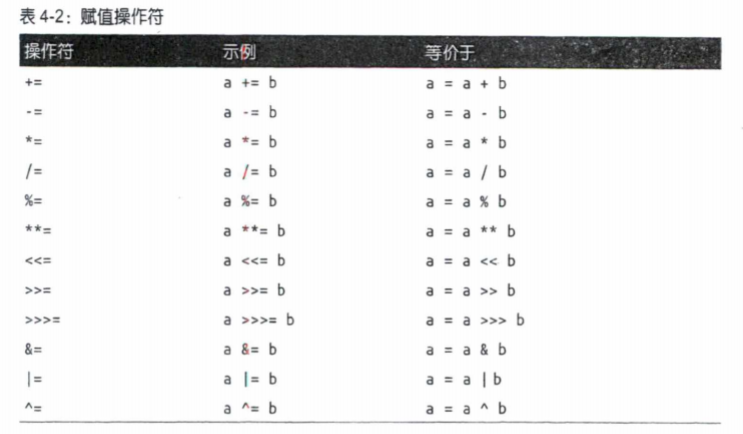``````a op=b
``````

（其中op是操作符）都等价于表达式：

``````a=a op b
``````

``````data[i++]*=2
data[i++]=data[i++]*2
``````
``````let data=[0,1,2,3]
let i=0
data[i++]*=2
data
=>(4) [0, 1, 2, 3]
let data=[0,1,2,3]
let i=0
data[i++]=data[i++]*2
data
=>(4) [2, 1, 2, 3]
``````

## 4.12 求值表达式

``````eval("3+2")             //=>5
``````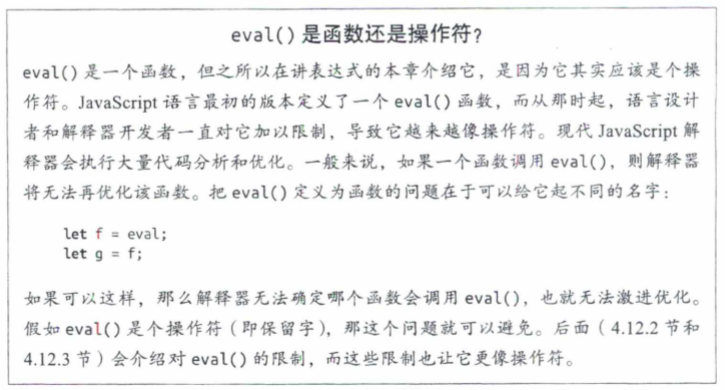### 4.12.1 eval()

eval()期待一个参数，如果给它传入任何非字符串值，它会简单地返回这个值。如果传入字符串，它会尝试把这个字符串当成JavaScript代码来解析，解析失败会抛出SyntaxError。如果解析字符串成功，它会求值代码并返回该字符串最后一个表达式或语句的值：如果最后一个表达式或语句没有值则返回undefined。如果求值字符串抛出异常，该异常会从调用eval()的地方传播出来。

``````eval("function f(){ return x+1; }");
``````

### 4.12.2 全局eval()

``````const geval=eval                    //使用另一个名字，实现全局求值
let x="global",y="global"
function f() {
let x="local";
eval("x +='changed';");
return x;
}

function g() {
let y="local";
geval("y+='changed';");
return y;
}

f()
=>"localchanged"
y
=>"globalchanged"
``````

## 4.13 其他操作符

### 4.13.1 条件操作符（?:）

``````greeting="hello"+(username?username:"there")
``````

``````greeting="hello"
}else{
greeting+="there"
}
``````

### 4.13.2 先定义（??）

==先定义操作符??求值其先定义的操作数，如果其左操作数不是null或undefined，就返回该值。否则，它会返回右操作数的值。==与&&或||操作符类似，??是短路的：它只有在第一个操作数求值为null或undefined才会求值第二个操作数。如果表达式a没有副效应，那么a??b等价于：

``````(a!==null&&a!==undefined)? a:b
``````

??是对||的一个有用的替代，适合选择先定义的操作数，而不是第一个为真值的操作数。尽管||名义上是个逻辑或操作符，习惯上也会使用它选择第一个非假值操作数：

``````//如果maxWith是真值，就使用它，否则，看看preferences
//对象。如果preferences里也没有真值，就使用硬编码的常量
let max=maxWidth||preferences.maxWith||500
``````

``````//如果maxWith有定义，就使用它，否则，看看preferences
//对象。如果preferences里也没有定义，就使用硬编码的常量
let max=maxWidth||preferences.maxWith||500
``````

??操作符与&&和||操作符类似，但优先级并不比它们更高或更低。如果表达式中混用了??和它们中的任何一个，必须使用圆括号说明先执行哪个操作：

``````(a ?? b) || c      //??先执行，然后执行||
a ?? (b || c)      //||先执行，然后执行??
a ?? b || c        //SyntaxError：必须有圆括号
``````

== ??操作符是ES2020定义的，在2020年初已经得到所有主流浏览器当前和预览版的支持。这个操作符的正式名称为“缺值合并”操作符，但我没有使用这个叫法。因为这个操作符会选择自己的一个操作数，但我并没有看到它会“合并”操作数。==

### 4.13.3 typeOf操作符

typeof是一个一元操作符，放在自己的操作数前面，这个操作数可以是任意类型。typeof操作符的值是一个字符串，表面操作数的类型。表4-3列出了所有JavaScript值在应用typeof操作符后得到的值。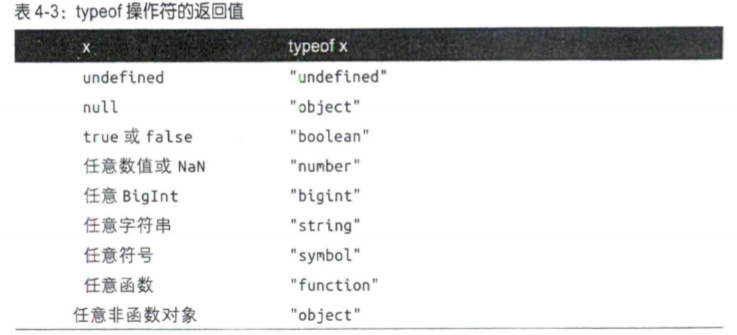``````//如果value是字符串，把它包含在引号中，否则把它转换成字符串
(typeof value === "string") ? "'" +value+ "'" :value.toString
``````

### 4.13.4 delete操作符

delete是一元操作符，尝试删除其操作数指定的对象属性或数组元素。与赋值、递增和递减操作符一样，使用delete通常也是为了发挥其属性删除的副效应，而不是使用它返回的值。来看几个例子：

``````let o={x:1,y:2}          //先定义一个对象
delete o.x
=>true                   //删除它的属性
"x" in o
=>false                  //这个属性不存在了
let a=[1,2,3]
delete a              //删除数组的最后一个元素
=>true
2 in a
=>false                  //数组元素2不存在了
a.length
=>3                      //但要注意，数组长度没有变化
``````

==注意，被删除的属性或数组元素不仅会被设置为undefined值。当删除一个属性是，这个属性就不复存在了。尝试读取不存在的属性会返回undefined，==但可以通过in操作符测试某个属性是否存在。删除某个数组元素会在数组中留下一个“坑”，并不改变数组的长度。结果数组是一个稀疏数组。

delete期待它的操作数是个左值，如果操作数不是左值，delete什么也不做，且返回true。否则，delete尝试删除指定的左值。如果删除成功则返回true，都是并非所有的属性都是可以删除的：不可配置属性（参见14.1节）就无法删除。

``````let o={x:1,y:2}
delete o.x
=>true                    //删除对象的一个属性；返回true
typeof o.x
=>"undefined"             //属性不存在；返回“undefined”
delete o.x
=>true                    //删除不存在的属性，返回true
delete 1
=>true                    //这样做毫无意义，但是会返回true
delete o
=>false                   //不能删除变量；返回false，或在严格模式下报TypeError
delete Object.prototype
=>false                   //不可删除的属性：返回false，或在严格模式下报TypeError
``````

### 4.13.5 await操作符

await是ES2017增加的，用于让JavaScript中的异步编程更自然。简单来说，await期待一个Promise对象（表示异步计算）作为其唯一操作数，可以让代码看起来像是在等待异步计算完成（但实际上它不会阻塞主线程，不会妨碍其他异步操作进行）。await操作符的值是Promise对象的兑现值。关键在于，await只能出现在已经通过async关键字声明为异步的函数中。

### 4.13.6 void操作符

void是一元操作符，出现在它的操作数前面，这个操作数可以是任意类型。void是个与众不同的操作符，用处不多：它求值自己的操作数，然后丢弃这个值并返回undefined。由于操作数的值会被丢弃，只有在操作数有副效应时才有必要使用void操作符。

void操作符太难解释，也很难给出一个实际的例子说明它的用法。一种情况是你要定义一个函数，这个函数什么也不返回，但却使用了箭头函数的简写语法，其中函数体是一个会被求值并返回的表达式。如果你只想对这个表达式求值，不想返回它的值，那最简单的方法就是用花括号把函数体包起来。此时，作为替代也可以使用void操作符：

``````let counter=0;
const increment=()=>void counter++;
increment()
=>undefined
counter
=>1
``````

### 4.13.7 逗号操作符(,)

``````i=0,j=1,k=2;
``````

``````i=0;j=1;k=2;
``````

``````//下面第一个逗号是let语句语法的一部分
//第二个逗号是逗号操作符，它让我们把两个表达式(i++与j--)
//放到了本来期待一个表达式的语句（for循环）中
for(let i=0,j=10;i<j;i++,j--){
console.log(i+j);
}
``````

17 0